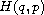Electron. J. Diff. Equ., Vol. 2014 (2014), No. 89, pp. 1-10.

### Hamiltonians representing equations of motion with damping due to friction Stephen Montgomery-Smith

Abstract:
Suppose thatis a Hamiltonian on a manifold M, and, the Rayleigh dissipation function, satisfies the same hypotheses as a Lagrangian on the manifold M. We provide a Hamiltonian framework that gives the equationThe method is to embed M into a larger framework where the motion drives a wave equation on the negative half line, where the energy in the wave represents heat being carried away from the motion. We obtain a version of Nother's Theorem that is valid for dissipative systems. We also show that this framework fits the widely held view of how Hamiltonian dynamics can lead to the arrow of time.''

Submitted January 23, 2014. Published April 2, 2014.
Math Subject Classifications: 70H25.
Key Words: Hamiltonian; Lagrangian; Rayleigh dissipation function; friction; Nother's Theorem.

Show me the PDF file (194 KB), TEX file, and other files for this article.Stephen Montgomery-Smith Department of Mathematics, University of Missouri Columbia, MO 65211, USA email: stephen@missouri.edu